# Sets Representation & Its Types Notes | Study Mathematics (Maths) Class 11 - JEE

## JEE: Sets Representation & Its Types Notes | Study Mathematics (Maths) Class 11 - JEE

The document Sets Representation & Its Types Notes | Study Mathematics (Maths) Class 11 - JEE is a part of the JEE Course Mathematics (Maths) Class 11.
All you need of JEE at this link: JEE

Introduction to Set

• We often deal with a group or a collection of objects, such as a collection of books, a collection of English alphabets, a class of students, a list of states in a country, a collection of coins, etc.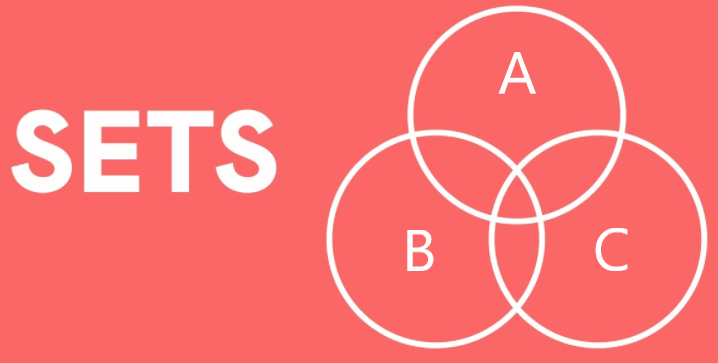• Set may be considered as a mathematical way of representingcollection or a group of objects.

Definition of Set

• “A set is a collection of well-defined objects.' In other words, a set is collection of defined and distinct objects. The objects of a set are called elements or members of the set. Further a set is always denoted by these brackets or braces { }.
Example: A = is a set of the letters of the word “FOLLOW’’
Then the set A will be a collection of the letters F, O, L, W.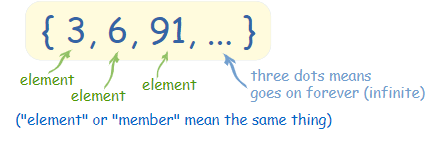Note: Here defined means existence of elements and distinct means there is no need to write repeated elements.

• The main property of a set is that it is well defined. This means that given any object, it must be clear whether that object is a member (element) of the set or not.
Generally, sets are named with the capital letters A, B, C, etc.
• The elements of a set are denoted by the small letters a, b, c, etc. and if a is an element of a set A then a ‘belongs to’ the set A where the phrase ‘belongs to’ is denoted by ∈ (which is also known as epsilon). Then we write a ∈ A. If a is not the element of A then we write a ∉ A.
Example: A is a set of natural numbers then 3 ∈ A and 0 ∉ A.

Some examples of sets in daily life are:

• Students of our classroom.
• Collection of animals of the world.
• Collection of fruits.
• Collection of vegetables.

Try yourself:Which of the following collections is a set?

Q.1. A collection of all-natural numbers less than 50.
Ans. It forms a set. The set will have the following elements.
A = {1, 2, 3, 4, 5, ................... 49}

Q.2. A collection of good hockey players in America
Ans. It doesn't form a set. Because the term "Good player" is vague and it is not well defined. However, a collection of players of hockey is a set.

Q.3. A collection of all girls in our class.
Ans. It forms a set. The elements of the above set are the names of those girls in the class.

Q.4. A collection of prime numbers less than 30
Ans. It forms a set. The set will have the following elements.
A = {2, 3, 5, 7, 11, 13, 17, 19, 23, 29}

Q.5. A collection of ten most talented mathematics teachers.
Ans. It doesn't form a set. Because the term "Most talented" is vague and is not well defined. However, a collection of mathematics teachers is a set.

In this chapter we will have frequent interaction with some sets, so we reserve some letters for these sets as listed below:
N: The set of natural numbers
Z: The set of integers
Z+: The set of all positive integers
Q: The set of all rational numbers
Q+: The set of all positive rational numbers
R: The set of all real numbers
R+: The set of all positive real numbers
C: The set of all complex numbers

➢ How to Represent a Set?

There are two methods to represent a set:

• Roster or tabular form.
• Set builder form.

1. Roster Form

In roster form, a set is described by listing the elements, separated by commas, within the braces.
Examples:
(i) A set of vowels of English alphabets is described by {a, e, i, o, u}
(ii) A set of whole numbers is described by {1, 2, 3, 4, 5, 6,………..}
(iii) A set even natural numbers is described by {2, 4, 6, 8, 10,……..}

2. Set Builder Form
To understand set builder form we can take the help of some examples:
As discussed above, A = {a, e, i, o, u}
In set builder form, A = {x : x is a vowel of English alphabets} or {x | x is a vowel of English alphabets}
B = {1, 2, 3, 4, 5, 6,……….}
In set builder form, B = {x : x is a natural number} or {x | x is a natural number} or {x : x ∈ N} C = {2, 4, 6, 8, 10,……..}
In set builder form
C = {x : x = 2n, n ∈ N}

Note: The set of real numbers cannot be represented by roster form. In set builder form, A = {x : x ∈ R}

Different Kind of Sets

1. Empty Set or Null Set: A set containing no elements is called the empty set or null set or void set.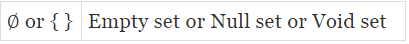So, it is denoted by { } or ∅.
Example: Consider the set A = {x : x < 1, x ∈ N}
There is no natural number which is less than 1.
Therefore, A = { }, or ∅.

Note: The concept of empty set plays a key role in the study of sets just like the role of the number zero in the study of the number system.

► Cardinal Number of a Set (Order of a set)
Cardinality or order of a set is defined as the number of elements that a set has. It is denoted by n(A) or c(A) or o(A) or |A| or  #A.
Example: A = {a, b, c} then n(A) = 3 and if A = { } or ∅ then n(A) = 0.

Try yourself:The cardinal number of the set {x: x ∈ Z, x= 1} is :

Q.1. Which of the following sets are empty?
(i) Set of counting numbers between 5 and 6.
(ii) Set of odd numbers between 7 and 19.
(iii) Set of odd numbers between 7 and 9.
(iv) Set of even numbers which are not divisible by 2.
(v) {0}
(vi) { }
(vii) {Prime numbers between 7 and 11}
(viii) {x | x ∈ N and 3< x < 4}
Ans. (i), (iii), (iv), (vi) & (viii) are empty sets.

Q.2. State, whether or not the following sets are empty:
(i) {1, 4, 7, 10, ………, 31}
(ii) {Month having more than 31 days}
(iii) {x | x2 = 0}
(iv) {x | x ∈ N and x < 1}
(v) {Prime numbers divisible by 2}
(vi) {Negative natural numbers}
(vii) {Women who are 5 meter tall}
(viii) {Men with four legs}
(ix) {Integers less than 5}
(x) {A week having 10 days}
(xi) {People who are 500 years old}
(xii) {Prime numbers between 17 and 23}
(xiii) {Set of even numbers, not divisible by 2}
(xiv) {Set of multiples of 3, which are more than 9 and less than 15}
Ans. Empty Sets: (ii), (iv), (vi), (vii), (viii), (x), (xi) & (xiii)
Not Empty Sets: (i), (iii), (v), (ix), (xii) & (xiv)

2. Finite Set: If the number of elements in a set is zero or finite, then the set is called a finite set.
Example:
(i) Consider the set A of natural numbers between 8 and 9.
There is no natural number between 8 and 9.
So, A = { } and n(A) = 0.
Hence, A is a finite set.
(ii) Consider the set X = {x : x is an integer and -1 ≤ x ≤ 2}
So, X = {-1, 0, 1, 2} and n(X) = 4
Hence, X is a finite set.

Note: The cardinal number of a finite set is finite.

3. Infinite Set: A set is said to be an infinite set if the number of elements in the set is not finite.
Example: Let W = The set of all whole numbers.
That is, W = {0, 1, 2, 3, ......................}
The set of all whole numbers contain infinite number of elements.
Hence, W is an infinite set.

Note: The cardinal number of an infinite set is not a finite number.

Try yourself:The set A = {x: x ∈ x= 16 and 2x = 6} equals:

Q.1. State, whether the given set is infinite or finite:
(i) {3, 5, 7, ….}
(ii) {1, 2, 3, 4}
(iii) {….., -3, -2, -1, 0, 1, 2}
(iv) {20, 30, 40, 50, ………., 200}
(v) {7, 14, 21, …………, 2401}
(vi) {0}
(vii) {∅}
(viii) {x | x is an even natural number less than 10, 000}
(ix) {All people in the world}
(x) {x | x is a prime number}
(xi) {x | x ∈ N and x > 10}
(xii) {….., -2, -1, 0}

Ans. Finite Sets: (ii), (iv), (v), (vi), (vii), (viii) & (ix)
Infinite Sets: (i), (iii), (x), (xi) & (xii)

Q.2. State, whether the following set is infinite or finite:
(i) Set of integers
(ii) {Multiples of 5}
(iii) {Fractions between 1 and 2}
(iv) {Numbers of people in India}
(v) Set of trees in the world
(vi) Set of prime numbers
(vii) Set of leaves on a tree
(viii) Set of children in all the schools of Delhi}
(ix) Set of seven natural numbers
(x) {……, -4, -, 2, 0, 2, 4, 6, 8}
(xi) {-12, -9, -6, -3, 0, 3, 6, ……}
(xii) {Number of points in a line segment 4 cm long}.

Ans. Finite Sets: (iv), (vii) & (viii)
Infinite Sets:
(i), (ii), (iii), (v), (vi), (ix), (x), (xi) & (xii)

Q.3. State, whether the following are finite sets, or infinite sets:
(i) {2, 4, 6, 8, …….., 800}
(ii) {-5, -4, -3, -2, …..}
(iii) {…., -5, -4, -3, -2,}
(iv) {Number of C.B.S.E. school in Delhi}
(v) {x : x is an integer between -60 and 60}
(vi) {No. of electrical appliances working in your house}
(vii) {x : x is a whole number greater than 20}
(viii) {x : x is a whole number less than 20}

Ans. Finite Sets: (i), (iv), (v), (vi) & (viii)
Infinite Sets: (ii), (iii) & (vii)

4. Singleton Set: A set containing only one element is called a singleton set.
Example: Consider the set A = {x : x is an integer and 1 < x < 3}
So, A = {2}. That is, A has only one element.
Hence, A is a singleton set.

Note: {0} is not null set. Because it contains one element. That is "0".

5. Equivalent Set: Two sets A and B are said to be equivalent if they have the same number of elements.

In other words, A and B are equivalent if n(A) = n(B).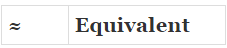"A and B are equivalent" is written as A ≈ B

For example:

Consider A = {1, 3, 5, 7, 9} and B = {a, e, i, o, u}

Here n(A) = n(B) =  5

Hence, A and B are equivalent sets.

6. Equal Sets: Two sets A and B are said to be equal if they contain exactly the same elements, regardless of order.

Otherwise, the sets are said to be unequal.

In other words, two sets A and B are said to be equal if

(i) Every element of A is also an element of B and

(ii) Every element of B is also an element of A.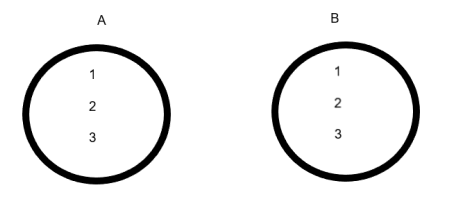Fig: A and B are Equal Sets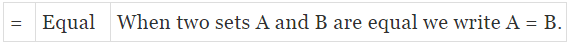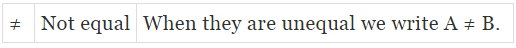Example: Consider A = {a, b, c, d} and B = {d, b, a, c}
Set A and set B contain exactly the same elements.
And also n(A) = n(B) = 4.
Hence, A and B are equal sets.
Note: If n(A) = n(B), then the two sets A and B need not be equal. Thus, equal sets are equivalent but equivalent sets need not be equal.

Q.1. State, if the given pairs of sets are equal sets or equivalent sets:
(a) {Natural numbers less than five} and {Letters of the word ‘BOAT’}
(b) {2, 4, 6, 8, 10} and {even natural numbers less than 12}
(c) {a, b, c, d} and {∆, ○, □, ∇}
(d) {Days of the week} and {Letters of the word ‘HONESTY}
(e) {1, 3, 5, 7, ……} and set of odd natural numbers.
(f) {Letters of the word ‘MEMBER’} and {Letters of the word ‘REMEMBER’}
(g) {Negative natural numbers} and {50th day of a month}
(h) {Even natural numbers} and {Odd natural numbers}

Ans.
(a) Equivalent
(b) Equal
(c) Equivalent
(d) Equivalent
(e) Equal
(f) Equal
(g) Equal
(h) Equivalent

The document Sets Representation & Its Types Notes | Study Mathematics (Maths) Class 11 - JEE is a part of the JEE Course Mathematics (Maths) Class 11.
All you need of JEE at this link: JEEUse Code STAYHOME200 and get INR 200 additional OFF

## Mathematics (Maths) Class 11

154 videos|171 docs|132 tests

Track your progress, build streaks, highlight & save important lessons and more!

,

,

,

,

,

,

,

,

,

,

,

,

,

,

,

,

,

,

,

,

,

;